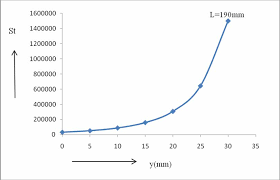## How to Calculate and Solve for Shielding Factor | Polymer & TextileThe image above represents shielding factor.

To compute for shielding factor, two essential parameter are needed and these parameters are coil radius (Rs) and shielding depth (L).

The formula for calculating the shielding factor:

ε = Rs / L

Where:

ε = Shielding Factor
L = Shielding Depth

Let’s solve an example;
Find the shielding factor when the coil radius is 11 and the shielding depth is 21.

This implies that;

Rs = Coil Radius = 11
L = Shielding Depth = 21

ε = Rs / L
ε = 11 / 21
ε = 0.52

Therefore, the shielding factor is 0.52.

Calculating for the Coil Radius when the Shielding Factor and the Shielding Depth is Given.

Rs = ε x L

Where;

ε = Shielding Factor
L = Shielding Depth

Let’s solve an example;
Given that the shielding factor is 30 and the shielding depth is 5. Find the coil radius?

This implies that;

ε = Shielding Factor = 30
L = Shielding Depth = 5

Rs = ε x L
Rs = 30 x 5
Rs = 150

Therefore, the coil radius is 150.